Document revision date: 15 July 2002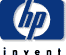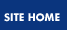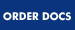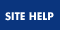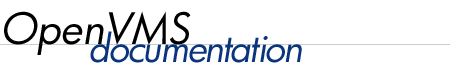## OpenVMS User's Manual

 Previous Contents Index

## 12.12 Understanding Symbol Substitution

In certain contexts, DCL uses a string of characters beginning with a letter as a symbol name or a lexical function. In these contexts, DCL tries to replace the symbol or lexical function with its value. Replacing a symbol with its current value is referred to as symbol substitution. If you use a symbol or lexical function in any other context, you must use a substitution operator to request symbol substitution.

DCL automatically evaluates symbols and lexical functions when they are used as follows:

• On the right side of an assignment (=) statement
• In an argument for a lexical function
• In a DEPOSIT, EXAMINE, IF, or WRITE command
• At the beginning of a command line when the string is not followed by an equal sign or a colon
• In the brackets on the left side of an assignment statement when you are performing substring substitution or numeric overlays (see Section 12.6.5)

In the following examples, the command interpreter uses any character string beginning with an alphabetic character as a symbol name and any string beginning with a number or with the radix operator (%) as a literal numeric value.

• In the following example, COUNT is automatically recognized and evaluated as a symbol:

 ```\$ TOTAL = COUNT + 1 ```

• In the second line of this example, the symbol QUERY is automatically evaluated when it is used with the F\$LENGTH function. In addition, the F\$LENGTH function is automatically evaluated because it is on the right side of an assignment statement:

 ```\$ QUERY = "Have we met before?" \$ LEN = F\$LENGTH(QUERY) + 5 \$ SHOW SYMBOL LEN LEN = 27 Hex = 0000001B Octal = 000033 ```

• In the following example, the IF command uses both A and B as symbol names and uses their current values:

 ```\$ IF A .EQ. B THEN WRITE SYS\$OUTPUT "DONE" ```

• In the second line of this example, the command interpreter automatically replaces PDEL with its current value and executes the resulting command:

 ```\$ PDEL = "DELETE SYS\$PRINT/ENTRY=" \$ PDEL 181 ```

• In the following example, DCL automatically defines the symbol BELL as the value of 7 and then assigns a new value based on the bracketed values on the left side of the assignment statement.

 ```\$ BELL = 7 \$ BELL[5,1] = 1 \$ SHOW SYMBOL BELL BELL = 39 Hex = 00000027 Octal = 00000000047 ```

### 12.12.1 Forced Symbol Substitution

To force substitution of a symbol that is not in one of the positions listed, enclose the symbol with apostrophes ('), as follows:

 ```\$ TYPE 'B' ```

To force substitution of a symbol within a quoted character string, precede that symbol with two apostrophes (') and follow it with a single apostrophe (') as follows:

 ```\$ T = "TYPE ''B'" ```

When processing a command line, DCL replaces symbols with their values in the following order:

• Forced substitution
From left to right, DCL replaces all strings delimited by apostrophes (or double apostrophes for strings within quotation marks). Symbols preceded by single apostrophes are translated iteratively; symbols preceded by double apostrophes are not.
• Automatic substitution
From left to right, DCL evaluates each value in the command line, executing it if it is a command and evaluating it if it is an expression. Symbols in expressions are replaced by their assigned values; this substitution is not iterative.

The following example demonstrates the effect of the order in which DCL substitutes symbols. First, the symbols PN, FILE1, and NUM are defined:

 ```\$ PN = "PRINT/NOTIFY" \$ FILE1 = "[BOLIVAR]TEST_CASE.TXT" \$ NUM = 1 ```

Given the preceding symbol definitions, the following commands print the file named [BOLIVAR]TEST_CASE.TXT:

 ```\$ FILE = "'FILE''NUM''" \$ PN 'FILE' ```

In the first command, forced substitution causes NUM to become 1, making FILE''NUM' become FILE1. If you enter the command SHOW SYMBOL FILE, you see that FILE = " 'FILE1' ".

The second command performs two substitutions. First, 'FILE' is substituted with 'FILE1'. 'FILE1' also requires substitution because it is enclosed in apostrophes ('). Automatic substitution causes FILE1 to become [BOLIVAR]TEST_CASE.TXT. This file name is then appended to the value of PN, which is PRINT/NOTIFY. The resulting string is as follows:

 ```\$ PRINT/NOTIFY [BOLIVAR]TEST_CASE.TXT ```

### 12.12.2 Symbol Substitution Operators

You can use a substitution operator to request symbol substitution in places where DCL does not usually perform it. DCL accepts two substitution operators:

• Apostrophe (')
• Ampersand (&)

The difference between these two operators is the time when the substitution occurs. Symbols preceded by apostrophes are substituted during the first phase of DCL command processing; symbols preceded by ampersands are substituted during the second phase. For more information on the phases of command processing, see Section 12.13.

The apostrophe (') is the most frequently used substitution operator. Use it to request symbol substitution when you use a symbol in place of a command parameter or qualifier. Use the apostrophes to request symbol substitution on the right side of a string assignment (:=) statement.

To request symbol substitution within a quoted character string, place two apostrophes before the symbol name and one apostrophe after it.

When you use apostrophes to request symbol substitution, you cannot continue the line (with the hyphen continuation character) in the middle of the value that is being substituted.

In the following example, the TYPE command requires a file specification. The apostrophes indicate that LIT is a symbol that must be evaluated. If you omit the apostrophes, DCL looks for a file called LIT.LIS (.LIS is the default file type for the TYPE command):

 ```\$ LIT = "LIGHT.BILLS" \$ TYPE 'LIT' ```

In the following example, the value for NAME is substituted so that FILE becomes REPORT.DAT:

 ```\$ NAME := REPORT \$ FILE := 'NAME'.DAT \$ SHOW SYMBOL FILE FILE = "REPORT.DAT" ```

In the following example, the current value of the symbol NAME is FRED:

 ```\$ MESSAGE = "Creating file ''NAME'.DAT" ```

Therefore, MESSAGE has the following value:

 ```Creating file FRED.DAT ```

The ampersand (&) is also a substitution operator that the command interpreter recognizes. In many cases, the apostrophe and the ampersand perform the same function. Ampersands are most effective as substitution operators when they are used with apostrophes to affect the order in which substitution is performed.

The action the command interpreter takes when a symbol is undefined depends on the context of the command. For more information, see Section 12.13.5.

In the first command shown here, the command interpreter replaces the symbol NAME with its current value during the first phase of command processing (scanning). The second command replaces the symbol NAME with its current value during the second phase of command processing (parsing). The result is the same, even though the methods are different:

 ```\$ TYPE 'NAME' \$ TYPE &NAME ```

In the following example, the ampersand (&) is used with apostrophes to affect the substitution order:

 ```\$ P1 = "FRED.DAT" \$ COUNT = 1 \$ TYPE &P'COUNT' ```

First, the command interpreter evaluates the symbol enclosed by apostrophes ('COUNT'). The result is as follows:

 ```TYPE &P1 ```

Second, the command interpreter evaluates the symbol preceded by an ampersand (P1). The result is as follows:

 ```TYPE FRED.DAT ```

In the following example, apostrophes are used with both P and COUNT:

 ```\$ TYPE 'P''COUNT' ```

Working left to right, the command interpreter attempts to evaluate P. Because P is not a defined symbol, DCL gives it a null value. Next, it evaluates the symbol COUNT. The result is as follows:

 ```TYPE 1 ```

In the following example, A is equated to the current value of B:

 ```\$ B = "MYFILE.DAT" \$ A = "&B" \$ TYPE 'A' ```

The ampersand (&) does not cause symbol substitution when it is used inside quotation marks (" "). Therefore, when the assignment is made, the value of B is not substituted. However, the TYPE command displays MYFILE.DAT. This occurs because the command interpreter first substitutes the value &B for A. Next, it substitutes MYFILE.DAT for the symbol &B. If you were to redefine B, the result of the TYPE command would change accordingly.

Observe the following rules for using ampersands:

• Place the ampersand before, but not after, the symbol name.
• An ampersand must follow a delimiter (any blank or special character).
• You cannot use ampersands to request substitution within character strings enclosed in quotation marks (" ").
• You cannot use ampersands to concatenate two or more symbol names.
• In general, do not use the ampersand for symbol substitution unless it is required to translate your symbols correctly.

## 12.13 The Three Phases of Command Processing

The command interpreter performs symbol substitution in three phases.

### 12.13.1 Phase 1: Command Input Scanning

In command input scanning (also called the lexical input phase), the command interpreter evaluates symbols preceded by apostrophes from left to right. Symbols that are preceded by single apostrophes are translated iteratively, as described in Phase 1 Substitution. Symbols preceded by two apostrophes are not translated iteratively.

### 12.13.2 Phase 2: Command Parsing

In the command parsing phase:

• The command interpreter analyzes the command line. It checks the first item on the line to see if it is a symbol. If it is, it is evaluated.
• The command interpreter evaluates symbols preceded by ampersands from left to right.

Symbol substitution during this phase is not iterative.

### 12.13.3 Phase 3: Expression Evaluation

During the expression evaluation phase:

• The command interpreter evaluates symbols that are preceded by the DEPOSIT, EXAMINE, IF, and WRITE commands.
• The command interpreter evaluates symbols within lexical functions.

Symbol substitution during this phase is not iterative.

Note that the command interpreter does not scan any lines that are read as input data by commands or programs executed within a command procedure. Therefore, the command interpreter does not perform symbol substitution within these data lines.

In the following example, the program AVERAGE reads 55, 57, and 9999 from SYS\$INPUT (the command input stream). These data lines are never read by the command interpreter. If you enter symbol names as input, they are not evaluated:

 ```\$ RUN AVERAGE 55 57 9999 ```

### 12.13.4 Repetitive and Iterative Substitution

Symbol substitution can be repetitive or iterative:

• Repetitive substitution results when more than one type of substitution occurs in a single command line.
• Iterative substitution occurs when the command interpreter examines a substituted value to see if the value itself is a symbol. Iterative substitution occurs only when symbols preceded by apostrophes are translated during the first phase of command processing.

When you use an apostrophe (') to request symbol substitution, the command interpreter performs iterative substitution during the first phase of command processing.

Substitution using apostrophes is not iterative when a symbol is included in a quoted character string.

In the following example, the substitution is iterative:

 ```\$ MAC = "5" \$ A = "'MAC'" \$ B = 'A' \$ SHOW SYMBOL B B = 5 Hex = 00000005 Octal = 00000000005 ```

After the statement B = 'A' the resulting value of the symbol B is 5 because:

• The symbol name A is enclosed in apostrophes, so it is replaced with its current value ('MAC').
• Because this value ('MAC') is also enclosed in apostrophes, the command interpreter replaces MAC with its current value (5).
• Because this value (5) has no apostrophes, the first phase of command processing is complete. No further substitution is required during the second or third phases. Therefore, 5 is the final value given to the symbol name B.

Note, however, what happens when you include A in a quoted character string:

 ```\$ B = "''A'" \$ SHOW SYMBOL B B = "'MAC'" ```

In this case, B has the value 'MAC'. The symbol name A is replaced only once because substitution is not iterative within quoted character strings.

The command interpreter performs iterative substitution automatically only when an apostrophe is in the command line. In some cases, you may want to nest command synonym definitions.

In the following example, when EXEC is processed, the command interpreter performs substitution only once:

 ```\$ MAC = "TYPE A.B" \$ EXEC = "'MAC'" \$ EXEC ```

The result is the string 'MAC'. The command interpreter displays an error message because it does not recognize MAC as a command. This error occurs because during the first phase of command processing, no substitution is performed (the string EXEC is not delimited by apostrophes). During the second phase, the string 'MAC' is substituted for EXEC because EXEC is the first value on the command line. This substitution is not iterative. Therefore, even though 'MAC' is delimited by apostrophes, no additional substitution is performed.

To use the command synonym EXEC correctly, enclose it in apostrophes:

 ```\$ 'EXEC' ```

In this case, the symbol EXEC is evaluated during the first phase of command processing. Because this substitution is iterative, ('MAC') is also evaluated and the string TYPE A.B is substituted.

When the command interpreter analyzes an expression in a command, any symbols specified in the expression are replaced only once. You can, however, force iterative substitution by using an apostrophe or an ampersand in the expression. When you force iteration in this way, you must remember the following:

• The command interpreter performs all substitutions requested by apostrophes and ampersands before the command string is executed.
• Commands that automatically perform symbol substitution do so after the first and second phases of command processing.

Note, however, that if substitution does not result in a valid symbol name, the command fails.

The following example shows iterative substitution in an IF command:

 ```\$ P1 = "FRED.DAT" \$ COUNT = 1 \$ IF P'COUNT' .EQS. "" THEN GOTO END ```

When the command interpreter scans this line, it replaces the symbol COUNT with its current value. The result is as follows:

 ```IF P1 .EQS. "" THEN GOTO END ```

Because this string has no apostrophes, the command interpreter does not perform any more substitution. However, when the IF command executes, it automatically evaluates the symbol name P1 and replaces it with its current value.

In the following example, the symbol name FILENAME is invalid:

 ```\$ FILENAME = "A.B" \$ IF 'FILENAME' .NES. "" THEN TYPE 'FILENAME' ```

The command interpreter replaces the symbol FILENAME with its current value (A.B). The result is as follows:

 ```IF A.B .NES. "" THEN TYPE A.B ```

When the IF command executes the command line, A.B is not a valid symbol and an error occurs. For this IF command to be processed correctly, omit the apostrophes, as follows:

 ```\$ IF FILENAME .NES. "" THEN TYPE 'FILENAME' ```

### 12.13.5 Undefined Symbols

If a symbol is not defined when it is used in a command line, the command interpreter either displays an error message or replaces the symbol with a null string, depending on the context. The rules are as follows:

• During the first and second phases of command processing, the command interpreter replaces all undefined symbols that are preceded by apostrophes or ampersands with null strings.
• During the third phase of command processing, if the command interpreter finds an undefined symbol, it displays a warning message and does not finish processing.

The following example shows how the command interpreter processes an undefined symbol that is preceded by an apostrophe:

 ```\$ FILE := MYFILE'FILE_TYPE' \$ SHOW SYMBOL FILE FILE = "MYFILE" \$ PRINT 'FILE' ```

When the symbol FILE is created, the symbol FILE_TYPE is replaced with its current value. If FILE_TYPE is not defined, the command interpreter replaces FILE_TYPE with a null string. The absence of a file type in the file specification causes the PRINT command to use the default file type .LIS. Thus, the file specification is interpreted as MYFILE.LIS.

In the following example, the expression is evaluated during the third phase of command processing:

 ```\$ A = 1 \$ C = A + B %DCL-W-UNDSYM, undefined symbol - check validity and spelling ```

The symbol B is undefined, so the command interpreter cannot evaluate the expression.

## 12.14 An Alternative to Using Symbols: Automatic Foreign Commands

You can also invoke a command procedure (.COM file type) or executable image (.EXE file type) from DCL level without defining a symbol for that procedure. Using automatic foreign commands, DCL can search a specific set of directories for a command procedure or executable image and run it automatically.

When you enter a command verb that is not a DCL symbol and that is not in the DCL command tables, the system usually displays the following message:

 ```DCL-W-IVVERB, unrecognized command verb - check validity and spelling ```

However, if the logical name DCL\$PATH is defined (and is not blank), DCL instead performs an RMS \$SEARCH for any file that contains the invalid verb in its file name and DCL\$PATH:.* as the default file specification.

If DCL finds a .COM or .EXE file, DCL will automatically execute that file with the rest of the command line as its parameters. (This behavior is similar to the PATH options found in DOS, UNIX, and other operating systems.)

In the following example, the DCL symbol SYSGEN is no longer needed. DCL looks in the SYS\$SYSTEM directory and finds SYSGEN.EXE. DCL acts like the symbol "SYSGEN" was defined as "\$SYS\$SYSTEM:SYSGEN" which causes the SYSGEN image to be activated as a foreign command.

 ```\$ SYSGEN %DCL-W-IVVERB, unrecognized command verb - check validity and spelling \SYSGEN\ \$ DEFINE DCL\$PATH SYS\$SYSTEM,SYS\$DISK:[]FOO \$ SYSGEN SHOW MAXPROCESSCNT Parameter Name Current Default Min. Max. Unit Dynamic -------------- ------- ------- ------- ------- ---- ------- MAXPROCESSCNT 157 32 12 8192 Processes ```

In the following example, SS does not need to be defined as "@SS.COM" because DCL will automatically search the SYS\$SYSTEM directory for SS.COM or SS.EXE. If that fails, DCL will search the current directory for SS.COM or SS.EXE.

 ```\$ TYPE SS.COM \$ SHOW SYMBOL/LOCAL/ALL \$ EXIT \$ SS "This is a parameter" P1 = "This is a parameter" P2 = "" P3 = "" P4 = "" P5 = "" P6 = "" P7 = "" P8 = "" \$ SS.EXE "This is a parameter" P1 = ".EXE" P2 = "This is a parameter" P3 = "" P4 = "" P5 = "" P6 = "" P7 = "" P8 = "" ```

In the example, DCL locates SS.COM and acts like "SS" had been a symbol defined as "@SS.COM". The command procedure is activated with the rest of the command line parsed as parameters. Note that "SS.EXE" does not invoke the image SS.EXE, but instead invokes SS.COM with two parameters, the first being the text string ".EXE". This is consistent with the way command parsing and symbol substitution is performed by the OpenVMS operating system.

 Previous Next Contents Index